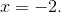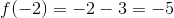# High School Math : Understanding the Definition of Limits

## Example Questions

### Example Question #1 : Limits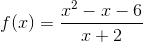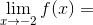Explanation:

A limit describes what-value a function approaches asapproaches a certain value (in this case,). The easiest way to find what-value a function approaches is to substitute the-value into the equation.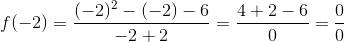Substitutingforgives us an undefined value (which is NOT the same thing as 0). This means the function is not defined at that point. However, just because a function is undefined at a point doesn't mean it doesn't have a limit. The limit is simply whichever value the function is getting close to.

One method of finding the limit is to try and simplify the equation as much as possible: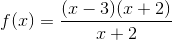As you can see, there are common factors between the numerator and the denominator that can be canceled out. (Remember, when you cancel out a factor from a rational equation, it means that the function has a hole -- an undefined point -- where that factor equals zero.)

After canceling out the common factors, we're left with: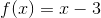Even though the domain of the original function is restricted (cannot equal), we can still substitute into this simplified equation to find the limit at